# Introduction

PKNCA considers two types of data grouping within data sets: the group and the interval. A group typically identifies a single subject given a single intervention type (a “treatment”) with a single analyte. An interval subsets a group by times within the group, and primary noncompartmental analysis (NCA) calculations are performed within an interval.

As a concrete example, consider the figure below shows the concentration-time profile of a study subject in a multiple-dose study. The group is all points in the figure, and the interval for the last day (144 to 168 hr) is the area with blue shading.

## Formula for concentration:
##  conc ~ time | treatment + ID
## Data are dense PK.
## With 1 subjects defined in the 'ID' column.
## Nominal time column is not specified.
##
## First 6 rows of concentration data:
##    study treatment ID time      conc   analyte exclude volume duration
##  Study 1     Trt 1  1    0 0.0000000 Analyte 1    <NA>     NA        0
##  Study 1     Trt 1  1    1 0.6140526 Analyte 1    <NA>     NA        0
##  Study 1     Trt 1  1    2 0.8100022 Analyte 1    <NA>     NA        0
##  Study 1     Trt 1  1    4 0.8425422 Analyte 1    <NA>     NA        0
##  Study 1     Trt 1  1    6 0.7771994 Analyte 1    <NA>     NA        0
##  Study 1     Trt 1  1    8 0.7052469 Analyte 1    <NA>     NA        0
# Plot the concentration-time data and the interval
ggplot(d_conc_multi, aes(x=time, y=conc)) +
geom_ribbon(data=d_conc$data, aes(ymax=conc, ymin=0), fill="skyblue") + geom_point() + geom_line() + scale_x_continuous(breaks=seq(0, 72, by=12)) + scale_y_continuous(limits=c(0, NA)) + labs(x="Time Since First Dose (hr)", y="Concentration\n(arbitrary units)")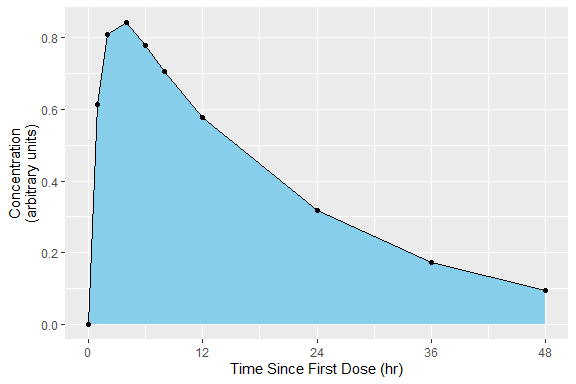intervals_manual <- data.frame( start=0, end=Inf, auclast=TRUE, aucinf.obs=TRUE ) print(intervals_manual) ## start end auclast aucinf.obs ## 1 0 Inf TRUE TRUE my.data <- PKNCAdata(d_conc, intervals=intervals_manual) ## Multiple Intervals More than one interval may be specified for the same subject or group of subjects by providing more than one row of interval specifications. In the figure below, the blue and green shaded regions indicate the first and second rows of the intervals, respectively. ## Formula for concentration: ## conc ~ time | treatment + ID ## Data are dense PK. ## With 1 subjects defined in the 'ID' column. ## Nominal time column is not specified. ## ## First 6 rows of concentration data: ## study treatment ID time conc analyte exclude volume duration ## Study 1 Trt 1 1 0 0.0000000 Analyte 1 <NA> NA 0 ## Study 1 Trt 1 1 1 0.6140526 Analyte 1 <NA> NA 0 ## Study 1 Trt 1 1 2 0.8100022 Analyte 1 <NA> NA 0 ## Study 1 Trt 1 1 4 0.8425422 Analyte 1 <NA> NA 0 ## Study 1 Trt 1 1 6 0.7771994 Analyte 1 <NA> NA 0 ## Study 1 Trt 1 1 8 0.7052469 Analyte 1 <NA> NA 0 # Plot the concentration-time data and the interval ggplot(d_conc_multi, aes(x=time, y=conc)) + geom_ribbon(data=d_conc_multi[d_conc_multi$time <= 24,],
aes(ymax=conc, ymin=0),
fill="skyblue") +
geom_ribbon(data=d_conc_multi[d_conc_multi$time >= 144,], aes(ymax=conc, ymin=0), fill="lightgreen") + geom_point() + geom_line() + scale_x_continuous(breaks=seq(0, 168, by=12)) + scale_y_continuous(limits=c(0, NA)) + labs(x="Time Since First Dose (hr)", y="Concentration\n(arbitrary units)")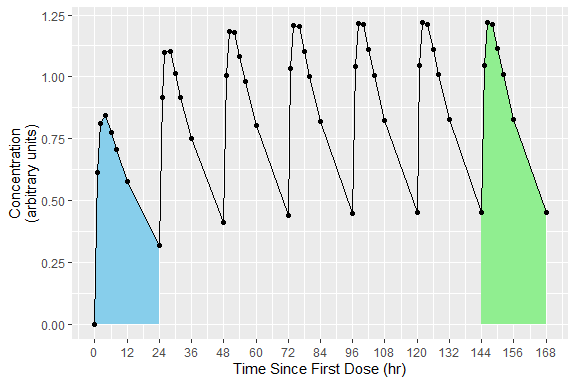intervals_manual <- data.frame( start=c(0, 144), end=c(24, 168), auclast=TRUE ) knitr::kable(intervals_manual) start end auclast 0 24 TRUE 144 168 TRUE my.data <- PKNCAdata(d_conc, intervals=intervals_manual) # Overlapping Intervals and Different Calculations by Interval In some scenarios, multiple intervals may be needed where some intervals overlap. There is no issue with an interval specification that has two rows with overlapping times; the rows are considered separately. In the example below, the 0-24 interval is shared between both the first and second (shaded blue-green). The example of overlapping intervals also illustrates that different calculations can be performed in different intervals. In this case, auclast is calculated in both intervals while aucinf.obs is only calculated in the 0-Inf interval. ## Formula for concentration: ## conc ~ time | treatment + ID ## Data are dense PK. ## With 1 subjects defined in the 'ID' column. ## Nominal time column is not specified. ## ## First 6 rows of concentration data: ## study treatment ID time conc analyte exclude volume duration ## Study 1 Trt 1 1 0 0.0000000 Analyte 1 <NA> NA 0 ## Study 1 Trt 1 1 1 0.6140526 Analyte 1 <NA> NA 0 ## Study 1 Trt 1 1 2 0.8100022 Analyte 1 <NA> NA 0 ## Study 1 Trt 1 1 4 0.8425422 Analyte 1 <NA> NA 0 ## Study 1 Trt 1 1 6 0.7771994 Analyte 1 <NA> NA 0 ## Study 1 Trt 1 1 8 0.7052469 Analyte 1 <NA> NA 0 # Use superposition to simulate multiple doses ggplot(d_conc$data, aes(x=time, y=conc)) +
geom_ribbon(data=d_conc$data, aes(ymax=conc, ymin=0), fill="lightgreen", alpha=0.5) + geom_ribbon(data=d_conc$data[d_conc$data$time <= 24,],
aes(ymax=conc, ymin=0),
fill="skyblue",
alpha=0.5) +
geom_point() + geom_line() +
scale_x_continuous(breaks=seq(0, 168, by=12)) +
scale_y_continuous(limits=c(0, NA)) +
labs(x="Time Since First Dose (hr)",
y="Concentration\n(arbitrary units)")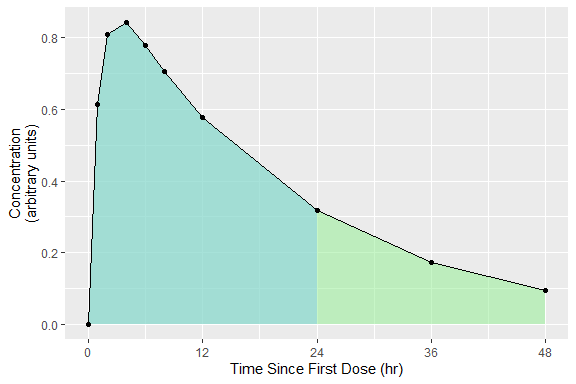intervals_manual <-
data.frame(
start=0,
end=c(24, Inf),
auclast=TRUE,
aucinf.obs=c(FALSE, TRUE)
)
knitr::kable(intervals_manual)
start end auclast aucinf.obs
0 24 TRUE FALSE
0 Inf TRUE TRUE
my.data <- PKNCAdata(d_conc, intervals=intervals_manual)

# Intervals with Duration

Some events have durations of times rather than instants in time associated with them. Two typical examples of duration data in NCA are intravenous infusions and urine or fecal sample collections. Inform PKNCA of durations with the duration argument to the PKNCAdose and PKNCAconc functions.

Durations data are selected based on both the beginning and ending of the duration existing within the interval.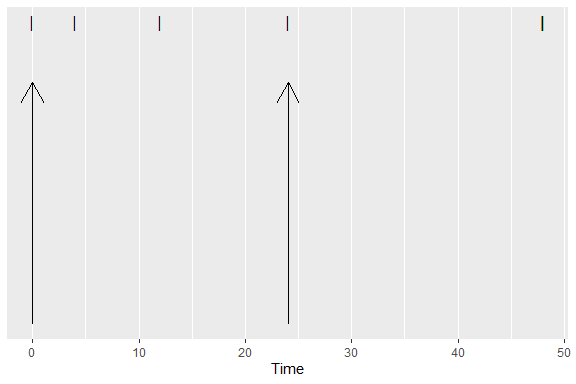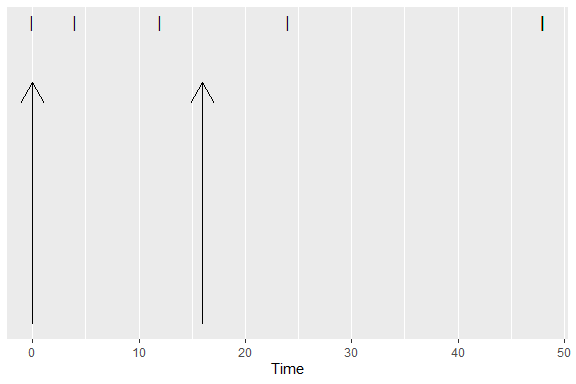# Parameters Available for Calculation in an Interval

The following parameters are available in an interval. For more information about the parameter, see the documentation for the function.

Parameter Name Unit Type Parameter Description Function for Calculation
adj.r.squared unitless The adjusted r^2 value of the half-life calculation See the parameter name half.life
ae amount The amount excreted (typically into urine or feces) pk.calc.ae
aucabove.predose.all auc The area under the concentration time the beginning of the interval to the last concentration above the limit of quantification plus the triangle from that last concentration to 0 at the first concentration below the limit of quantification, with a concentration subtracted from all concentrations and values below zero after subtraction set to zero pk.calc.aucabove
aucabove.trough.all auc The area under the concentration time the beginning of the interval to the last concentration above the limit of quantification plus the triangle from that last concentration to 0 at the first concentration below the limit of quantification, with a concentration subtracted from all concentrations and values below zero after subtraction set to zero pk.calc.aucabove
aucall auc The area under the concentration time curve from the beginning of the interval to the last concentration above the limit of quantification plus the triangle from that last concentration to 0 at the first concentration below the limit of quantification pk.calc.auc.all
aucall.dn auc_dosenorm Dose normalized aucall pk.calc.dn
aucinf.obs auc The area under the concentration time curve from the beginning of the interval to infinity with extrapolation to infinity from the observed Clast pk.calc.auc.inf.obs
aucinf.obs.dn auc_dosenorm Dose normalized aucinf.obs pk.calc.dn
aucinf.pred auc The area under the concentration time curve from the beginning of the interval to infinity with extrapolation to infinity from the predicted Clast pk.calc.auc.inf.pred
aucinf.pred.dn auc_dosenorm Dose normalized aucinf.pred pk.calc.dn
aucint.all auc The area under the concentration time curve in the interval extrapolating from Tlast to infinity with the triangle from Tlast to the next point and zero thereafter (matching AUCall) pk.calc.aucint.all
aucint.all.dose auc The area under the concentration time curve in the interval extrapolating from Tlast to infinity with the triangle from Tlast to the next point and zero thereafter (matching AUCall) with dose-aware interpolation/extrapolation of concentrations pk.calc.aucint.all
aucint.inf.obs auc The area under the concentration time curve in the interval extrapolating from Tlast to infinity with zeros (matching AUClast) pk.calc.aucint.inf.obs
aucint.inf.obs.dose auc The area under the concentration time curve in the interval extrapolating from Tlast to infinity with zeros (matching AUClast) with dose-aware interpolation/extrapolation of concentrations pk.calc.aucint.inf.obs
aucint.inf.pred auc The area under the concentration time curve in the interval extrapolating from Tlast to infinity with the triangle from Tlast to the next point and zero thereafter (matching AUCall) pk.calc.aucint.inf.pred
aucint.inf.pred.dose auc The area under the concentration time curve in the interval extrapolating from Tlast to infinity with the triangle from Tlast to the next point and zero thereafter (matching AUCall) with dose-aware interpolation/extrapolation of concentrations pk.calc.aucint.inf.pred
aucint.last auc The area under the concentration time curve in the interval extrapolating from Tlast to infinity with zeros (matching AUClast) pk.calc.aucint.last
aucint.last.dose auc The area under the concentration time curve in the interval extrapolating from Tlast to infinity with zeros (matching AUClast) with dose-aware interpolation/extrapolation of concentrations pk.calc.aucint.last
aucivall auc The AUCall calculated with back-extrapolation for intravenous dosing using extrapolated C0 pk.calc.auciv
aucivinf.obs auc The AUCinf,obs calculated with back-extrapolation for intravenous dosing using extrapolated C0 pk.calc.auciv
aucivinf.pred auc The calculated with back-extrapolation for intravenous dosing using extrapolated C0 pk.calc.auciv
aucivint.all auc The AUCint,all calculated with back-extrapolation for intravenous dosing using extrapolated C0 pk.calc.auciv
aucivint.last auc The AUCint,last calculated with back-extrapolation for intravenous dosing using extrapolated C0 pk.calc.auciv
aucivlast auc The AUClast calculated with back-extrapolation for intravenous dosing using extrapolated C0 pk.calc.auciv
aucivpbextall % The back-extrapolation percent for intravenous dosing based on AUCall pk.calc.auciv_pbext
aucivpbextinf.obs % The back-extrapolation percent for intravenous dosing based on AUCinf,obs pk.calc.auciv_pbext
aucivpbextinf.pred % The back-extrapolation percent for intravenous dosing based on AUCinf,pred pk.calc.auciv_pbext
aucivpbextint.all % The back-extrapolation percent for intravenous dosing based on AUCint,all pk.calc.auciv_pbext
aucivpbextint.last % The back-extrapolation percent for intravenous dosing based on AUCint,last pk.calc.auciv_pbext
aucivpbextlast % The back-extrapolation percent for intravenous dosing based on AUClast pk.calc.auciv_pbext
auclast auc The area under the concentration time curve from the beginning of the interval to the last concentration above the limit of quantification pk.calc.auc.last
auclast.dn auc_dosenorm Dose normalized auclast pk.calc.dn
aucpext.obs % Percent of the AUCinf that is extrapolated after Tlast calculated from the observed Clast pk.calc.aucpext
aucpext.pred % Percent of the AUCinf that is extrapolated after Tlast calculated from the predicted Clast pk.calc.aucpext
aumcall aumc The area under the concentration time moment curve from the beginning of the interval to the last concentration above the limit of quantification plus the moment of the triangle from that last concentration to 0 at the first concentration below the limit of quantification pk.calc.aumc.all
aumcall.dn aumc_dosenorm Dose normalized aumcall pk.calc.dn
aumcinf.obs aumc The area under the concentration time moment curve from the beginning of the interval to infinity with extrapolation to infinity from the observed Clast pk.calc.aumc.inf.obs
aumcinf.obs.dn aumc_dosenorm Dose normalized aumcinf.obs pk.calc.dn
aumcinf.pred aumc The area under the concentration time moment curve from the beginning of the interval to infinity with extrapolation to infinity from the predicted Clast pk.calc.aumc.inf.pred
aumcinf.pred.dn aumc_dosenorm Dose normalized aumcinf.pred pk.calc.dn
aumclast aumc The area under the concentration time moment curve from the beginning of the interval to the last concentration above the limit of quantification pk.calc.aumc.last
aumclast.dn aumc_dosenorm Dose normalized aumclast pk.calc.dn
c0 conc Initial concentration after an IV bolus pk.calc.c0
cav conc The average concentration during an interval pk.calc.cav
cav.dn conc_dosenorm Dose normalized cav pk.calc.dn
ceoi conc Concentration at the end of infusion pk.calc.ceoi
cl.all clearance Clearance or observed oral clearance calculated with AUCall pk.calc.cl
cl.last clearance Clearance or observed oral clearance calculated to Clast pk.calc.cl
cl.obs clearance Clearance or observed oral clearance calculated with observed Clast pk.calc.cl
cl.pred clearance Clearance or observed oral clearance calculated with predicted Clast pk.calc.cl
clast.obs conc The last concentration observed above the limit of quantification pk.calc.clast.obs
clast.obs.dn conc_dosenorm Dose normalized clast.obs pk.calc.dn
clast.pred conc The concentration at Tlast as predicted by the half-life See the parameter name half.life
clast.pred.dn conc_dosenorm Dose normalized clast.pred pk.calc.dn
clr.last renal_clearance The renal clearance calculated using AUClast pk.calc.clr
clr.obs renal_clearance The renal clearance calculated using AUCinf,obs pk.calc.clr
clr.pred renal_clearance The renal clearance calculated using AUCinf,pred pk.calc.clr
cmax conc Maximum observed concentration pk.calc.cmax
cmax.dn conc_dosenorm Dose normalized cmax pk.calc.dn
cmin conc Minimum observed concentration pk.calc.cmin
cmin.dn conc_dosenorm Dose normalized cmin pk.calc.dn
cstart conc The predose concentration pk.calc.cstart
ctrough conc The trough (end of interval) concentration pk.calc.ctrough
ctrough.dn conc_dosenorm Dose normalized ctrough pk.calc.dn
deg.fluc % Degree of fluctuation pk.calc.deg.fluc
end time Ending time of the interval (potentially infinity) (none)
f fraction Bioavailability or relative bioavailability pk.calc.f
fe fraction The fraction of the dose excreted pk.calc.fe
half.life time The (terminal) half-life pk.calc.half.life
kel.iv.last inverse_time Elimination rate (as calculated from the intravenous MRTlast) pk.calc.kel
kel.iv.obs inverse_time Elimination rate (as calculated from the intravenous MRTobs) pk.calc.kel
kel.iv.pred inverse_time Elimination rate (as calculated from the intravenous MRTpred) pk.calc.kel
kel.last inverse_time Elimination rate (as calculated from the MRT using AUClast) pk.calc.kel
kel.obs inverse_time Elimination rate (as calculated from the MRT with observed Clast) pk.calc.kel
kel.pred inverse_time Elimination rate (as calculated from the MRT with predicted Clast) pk.calc.kel
lambda.z inverse_time The elimination rate of the terminal half-life See the parameter name half.life
lambda.z.n.points count The number of points used for the calculation of half-life See the parameter name half.life
lambda.z.time.first time The first time point used for the calculation of half-life See the parameter name half.life
mrt.iv.last time The mean residence time to the last observed concentration above the LOQ correcting for dosing duration pk.calc.mrt.iv
mrt.iv.obs time The mean residence time to infinity using observed Clast correcting for dosing duration pk.calc.mrt.iv
mrt.iv.pred time The mean residence time to infinity using predicted Clast correcting for dosing duration pk.calc.mrt.iv
mrt.last time The mean residence time to the last observed concentration above the LOQ pk.calc.mrt
mrt.md.obs time The mean residence time with multiple dosing and nonlinear kinetics using observed Clast pk.calc.mrt.md
mrt.md.pred time The mean residence time with multiple dosing and nonlinear kinetics using predicted Clast pk.calc.mrt.md
mrt.obs time The mean residence time to infinity using observed Clast pk.calc.mrt
mrt.pred time The mean residence time to infinity using predicted Clast pk.calc.mrt
ptr fraction Peak-to-Trough ratio (fraction) pk.calc.ptr
r.squared unitless The r^2 value of the half-life calculation See the parameter name half.life
span.ratio fraction The ratio of the half-life to the duration used for half-life calculation See the parameter name half.life
sparse_auclast auc For sparse PK sampling, the area under the concentration time curve from the beginning of the interval to the last concentration above the limit of quantification pk.calc.sparse_auclast
start time Starting time of the interval (none)
swing % Swing relative to Cmin pk.calc.swing
tfirst time Time of the first concentration above the limit of quantification pk.calc.tfirst
thalf.eff.iv.last time The effective half-life (as determined from the intravenous MRTlast) pk.calc.thalf.eff
thalf.eff.iv.obs time The effective half-life (as determined from the intravenous MRTobs) pk.calc.thalf.eff
thalf.eff.iv.pred time The effective half-life (as determined from the intravenous MRTpred) pk.calc.thalf.eff
thalf.eff.last time The effective half-life (as determined from the MRTlast) pk.calc.thalf.eff
thalf.eff.obs time The effective half-life (as determined from the MRTobs) pk.calc.thalf.eff
thalf.eff.pred time The effective half-life (as determined from the MRTpred) pk.calc.thalf.eff
time_above time Time above a given concentration pk.calc.time_above
tlag time Lag time pk.calc.tlag
tlast time Time of the last concentration observed above the limit of quantification pk.calc.tlast
tmax time Time of the maximum observed concentration pk.calc.tmax
vd.obs volume Apparent observed volume of distribution calculated with observed Clast pk.calc.vd
vd.pred volume Apparent observed volume of distribution calculated with predicted Clast pk.calc.vd
vss.iv.last volume The steady-state volume of distribution with intravenous infusion calculating through Tlast pk.calc.vss
vss.iv.obs volume The steady-state volume of distribution with intravenous infusion using observed Clast pk.calc.vss
vss.iv.pred volume The steady-state volume of distribution with intravenous infusion using predicted Clast pk.calc.vss
vss.last volume The steady-state volume of distribution calculating through Tlast pk.calc.vss
vss.md.obs volume The steady-state volume of distribution for nonlinear multiple-dose data using observed Clast pk.calc.vss
vss.md.pred volume The steady-state volume of distribution for nonlinear multiple-dose data using predicted Clast pk.calc.vss
vss.obs volume The steady-state volume of distribution using observed Clast pk.calc.vss
vss.pred volume The steady-state volume of distribution using predicted Clast pk.calc.vss
vz.obs volume The terminal volume of distribution using observed Clast pk.calc.vz
vz.pred volume The terminal volume of distribution using predicted Clast pk.calc.vz